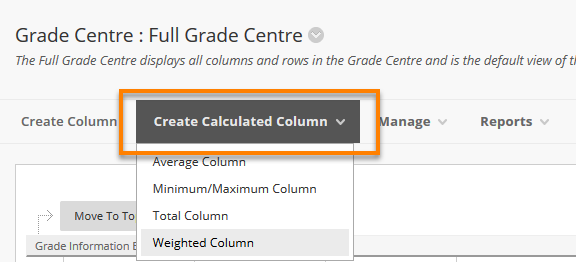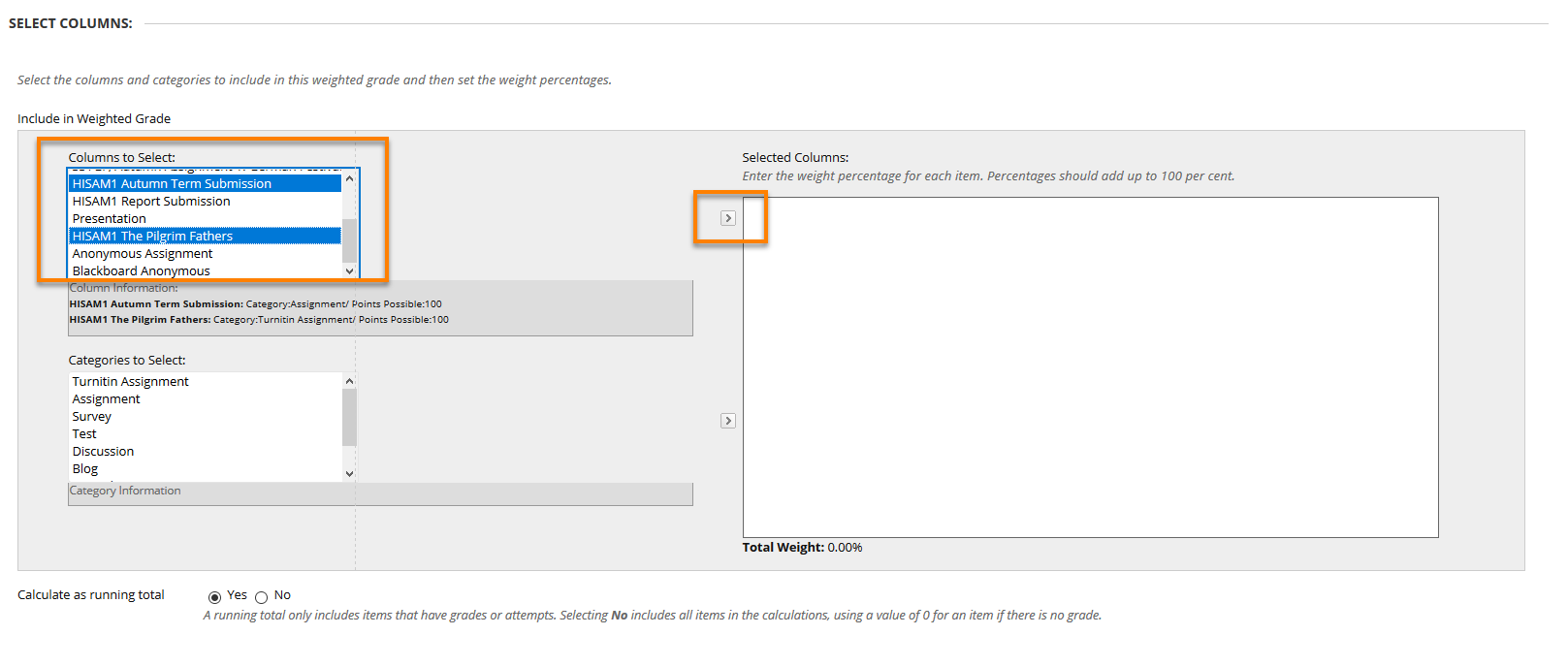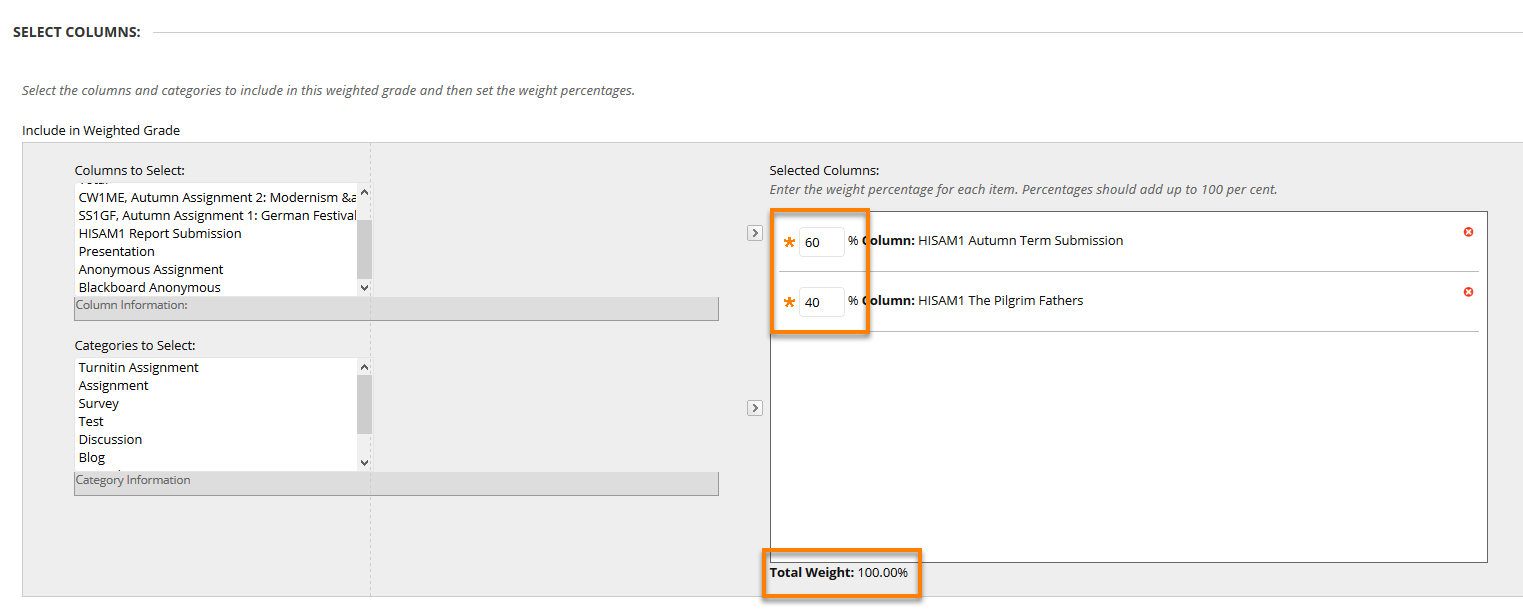# Blackboard: Grade Centre – Using Calculated Columns

## Introduction

In the Grade Centre, you can calculate marks by combining multiple columns to attain performance results, such as

• course averages
• final marks based on a weighted scale (combining two or more different marks together)
• total points.

These are called calculated columns. You can display these columns to students so they can view performance results, or you can display them only in the Grade Centre for your use.

## Types of Calculated Column

The available calculated columns include the following:

• Total
This simply generates a mark from the total points awarded to the student from all assessments on the course. The mark is not weighted, and it is not shown as a percentage of the maximum number of points available. You can edit which Grade Columns are included in the Total column, but in most cases it is best to hide this column from student view (see separate TEL user guide).
• Average
A calculated column that displays the average for a selected number of columns.
• Minimum/Maximum
A calculated column that displays either the minimum or maximum mark for a selection of columns.
• Weighted
A calculated column that generates a mark based on the result of selected columns and categories, and their respective percentages. This is likely to be the most useful column to use.

New courses contain by default a Total column and a Weighted Total column.  You can edit these, or create your own additional columns.

## Creating and using a Weighted Column

A Weighted Column calculates and displays a mark based for a selected number of Columns, based upon each column’s respective worth of the total mark. To be included in the calculation, columns must have a numerical score – columns with text as the primary display (e.g. A, B, C) cannot be included in a weighted mark.

A Weighted Column can include:

• All Grade Columns in Marking Period: All Grade Columns that have been added to a Marking Period.
• Selected Categories: Where assessments have been assigned to a Category, you can include any or all Categories in the calculation.
N.B. Columns in Categories which are set not to be included in Grade Centre calculations are ignored when calculating the Average.

### Weighted Column Examples

An Instructor may create a Weighted Grade which will be the student’s final mark, based on a combination of various assignments, each with a different weighting.

e.g. Total mark = (Assignment 1 = 20%) + (Assignment 2 = 30%) + (Assignment 3 = 50%)

Instructors can create any number of Weighted Grade columns, including Weighted Grade columns that include other Weighted Grade columns. For example:

Weighted Column 1 = (Assignment 1 = 40%) + (Assignment 2 = 60%)

Weighted Column 2 = (Assignment 3 = 20%) + (Assignment 2 = 80%)

Total mark = (Weighted Column 1 = 40%) + (Weighted Column 2 = 60%)

### Creating a Weighted Column

To create any type of calculated column, go into the Full Grade Centre, click on the Create Calculated Column button from the top menu then select the type of column you want to create.### Step 1: Add basic Column information

On the Create Weighted Column page

• Provide a Column Name (required field).
• You can enter an alternative Grade Centre Display Name, perhaps an abbreviated version of the full assignment name – this is what appears as the Column header in the Grade Centre.
• A Description is optional but helps Instructors and other markers identify the column.
• The Primary Display option determines how the mark displays in the Grade Centre and to students via My Grades. Selection options are Score, Text, Percentage, Letter and Complete/Incomplete.
• The Secondary Display option applies only to how the mark will display in the Grade Centre, where any Secondary Display is denoted by parentheses.

### Step 2: Select Columns to include

1.       Highlight one or more columns on the left, and click the arrow to move these to the list of Selected Columns on the right.
(To remove a previously selected Category or Column from the calculation, click the red “x”).2.      Enter a percentage that reflects the weight for each selected Column. The percentages of all Columns should add up to 100 percent.You can also create a calculated column by selecting a Category, which will give additional options on how to calculate marks. For more information please click on the More Help link at the top of the page.

3.       It is normally best to select No for the Calculate as running total option.
A Running Total calculates the weighted total by including only those Grade Columns where attempts have been marked. Selecting No for this option means that assessments where no submission has been made, or which have not yet been marked, will have a score of zero. This might appear to give artificially low scores while the module is running, but does give a more reliable indicator of students’ progress towards an overall module score.

```Please note: for Weighted Total columns created by the EMA Integration, it is important to leave 'Calculate as Running Total' to the default value of Yes.```

4.      Glance over the Options section and configure as required – in particular you may well want to say No to ‘Show this Column to Students’ while marking and moderation is in progress.

5.       Press Submit